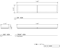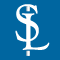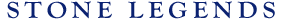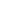HOMEPRODUCTSIDEA CENTERSERVICESTECHNICALABOUT USHello Guest Login  |  Become Member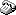800-398-1199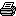214-398-1293MapEmail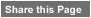Click to Share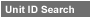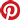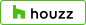Select which variables you would like to search by.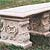Type DescriptionPrimary ViewPrimary ModifierUnit NameView Definitions

View Definitions

View DefinitionsX-Dim Y-Dim Z-Dim XX-Dim YY-Dim ZZ-Dim R-Dim D-Dim P-Dim
 Click column heading to re-sort results list.Product Name Primary View Modifier Pri Dimx  y  z Alt Dimxx  yy  zz Alt2 Dimr  d  p E\$timated Thumbnail Meyerson Bench 2 Benches Rectangular X = 18-3/8" Y = 60"Z = 14" XX = n/a YY = n/aZZ = n/a R = n/a D = n/aP = n/a \$ 438.00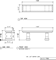Meyerson Bench1 Benches Rectangular X = 14" Y = 48"Z = 14" XX = n/a YY = n/aZZ = n/a R = n/a D = n/aP = n/a \$ 400.00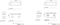Triste Bench1 Benches Radius DecorativeLegs X = 18" Y = 51"Z = 16" XX = n/a YY = n/aZZ = n/a R = 176" D = n/aP = n/a \$ 790.00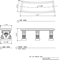Triste Bench2 Benches Radius DecorativeLegs X = 18" Y = 72"Z = 16" XX = n/a YY = n/aZZ = n/a R = 30" D = n/aP = n/a \$ 790.00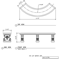Triste Bench3 Benches Rectangular DecorativeLegs X = 18" Y = 48"Z = 16" XX = n/a YY = n/aZZ = n/a R = n/a D = n/aP = n/a \$ 600.00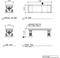Triste Bench4 Benches Radius DecorativeLegs X = 17-5/8" Y = 46-15/16"Z = 16" XX = n/a YY = n/aZZ = n/a R = 48-1/2" D = n/aP = n/a \$ 575.00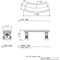Wall Bench Benches Rectangular X = 16" Y = 84"Z = 3" XX = n/a YY = n/aZZ = n/a R = n/a D = n/aP = n/a \$ 140.00